# NCERT Solutions for Class 8 Maths Chapter 7 Cubes and Cube Roots Ex 7.1

NCERT Solutions for Class 8 Maths Chapter 7 Cubes and Cube Roots Ex 7.1 are part of NCERT Solutions for Class 8 Maths. Here we have given NCERT Solutions for Class 8 Maths Chapter 7 Cubes and Cube Roots Ex 7.1.

 Board CBSE Textbook NCERT Class Class 8 Subject Maths Chapter Chapter 7 Chapter Name Cubes and Cube Roots Exercise Ex 7.1 Number of Questions Solved 4 Category NCERT Solutions

## NCERT Solutions for Class 8 Maths Chapter 7 Cubes and Cube Roots Ex 7.1

Question 1.
Which of the following numbers are not perfect cubes?
(i) 216
(ii) 128
(iii) 1000
(iv) 100
(v) 46656
Solution.
(i) 216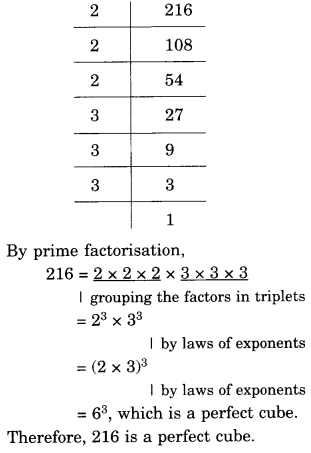(ii) 128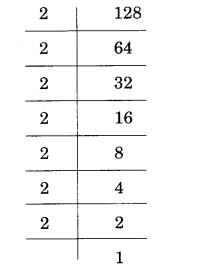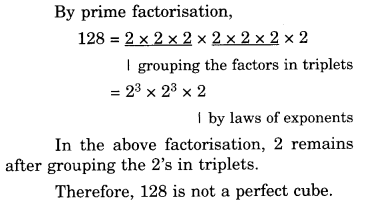(iii) 1000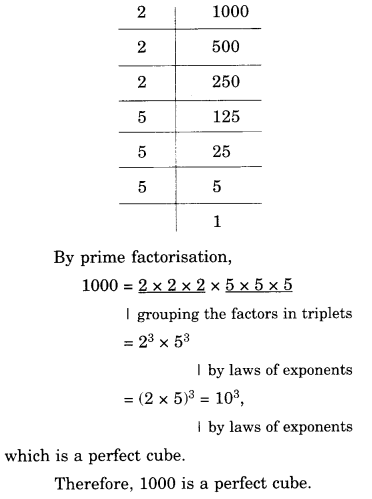(iv) 100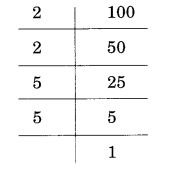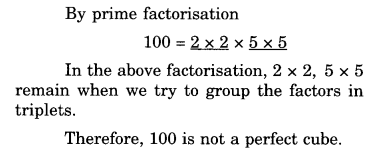(v) 46656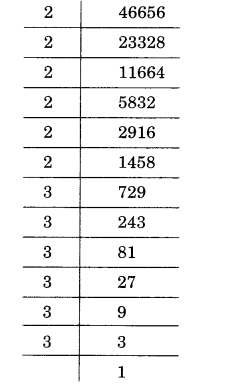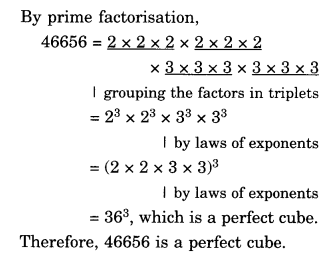Question 2.
Find the smallest number by which each of the following numbers must be multiplied to obtain a perfect cube.
(i) 243
(ii) 256
(iii) 72
(iv) 675
(v) 100
Solution.
(i) 243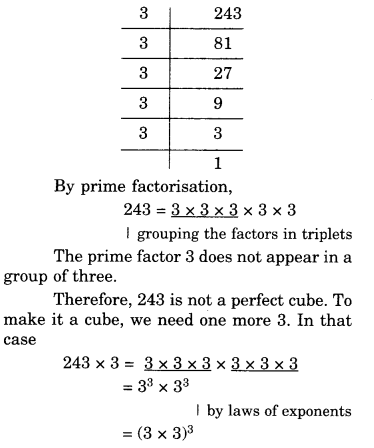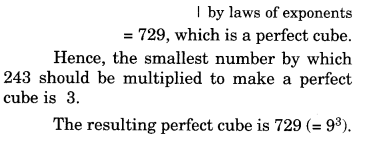(ii) 256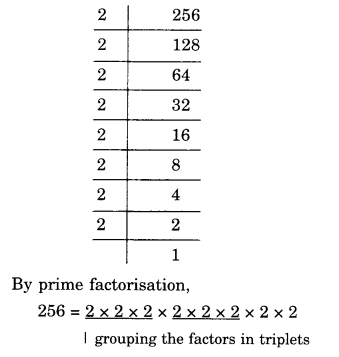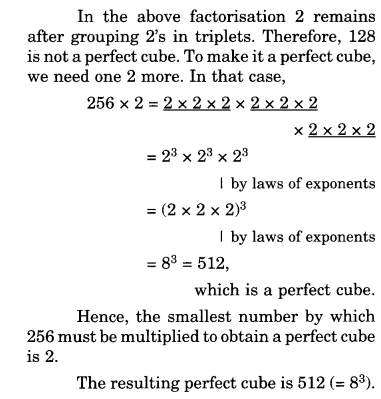(iii) 72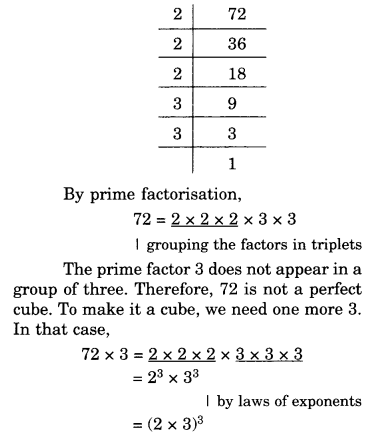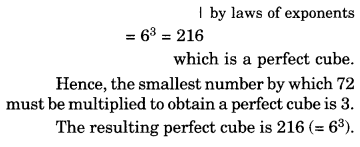(iv) 675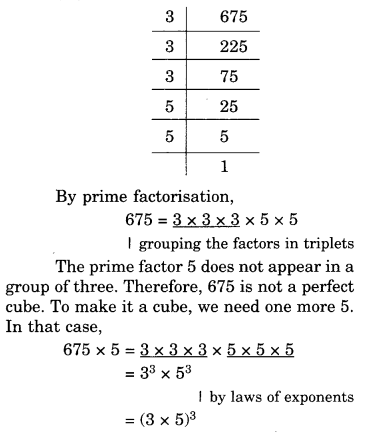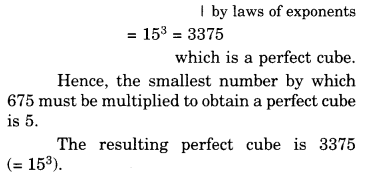(v) 100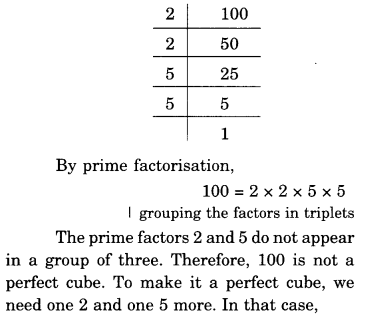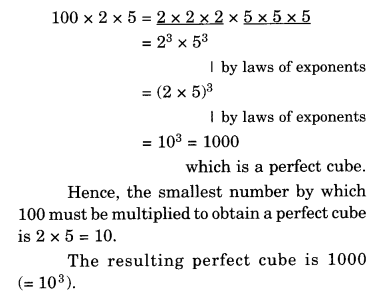Question 3.
Find the smallest number by which each of the following numbers must be divided to obtain a perfect cube.
(i) 81
(ii) 128
(iii) 135
(iv) 192
(v) 704
Solution.
(i) 81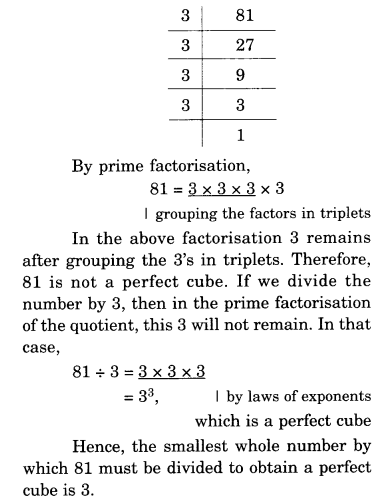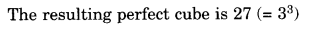(ii) 128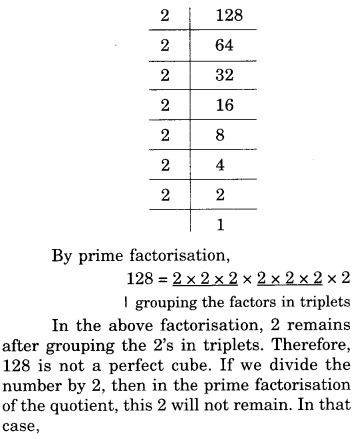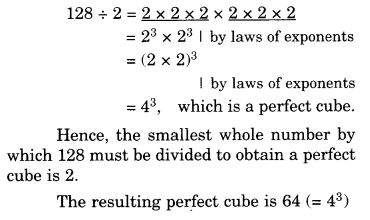(iii) 135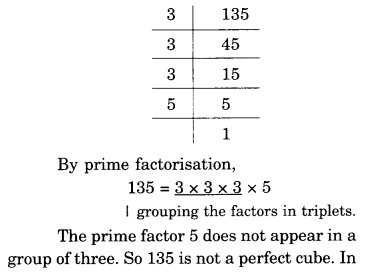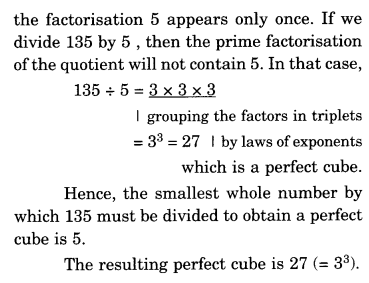(iv) 192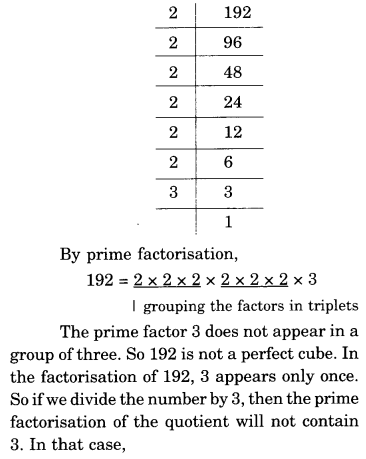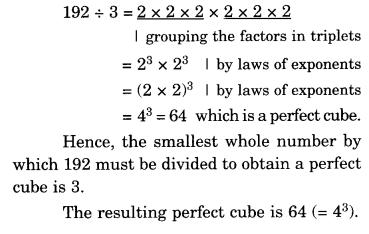(v) 704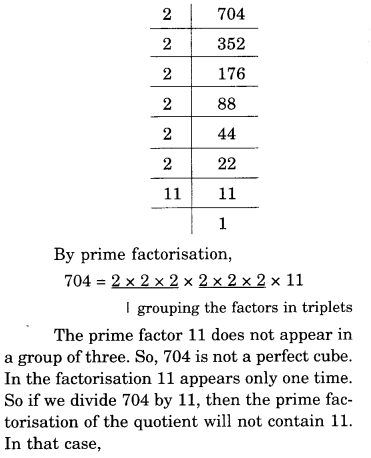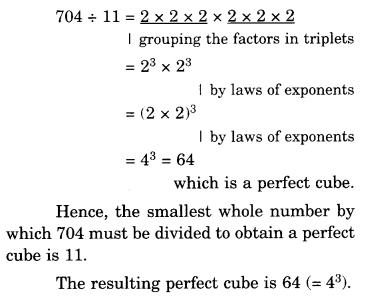Question 4.
Parikshit makes a cuboid of plasticine of sides 5 cm, 2 cm, 5 cm. How many such cuboids will he need to form a cube?
Solution.
Volume of a cuboid = 5 x 2 x 5 $${ cm }^{ 3 }$$.
Since there is only one 2 and only two 5’s in the prime factorization, so, we need 2 x 2 x 5, i.e., 20 to make a perfect cube. Therefore, we need 20 such cuboids to make a cube.

We hope the NCERT Solutions for Class 8 Maths Chapter 7 Cubes and Cube Roots Ex 7.1 help you. If you have any query regarding NCERT Solutions for Class 8 Maths Chapter 7 Cubes and Cube Roots Ex 7.1, drop a comment below and we will get back to you at the earliest.